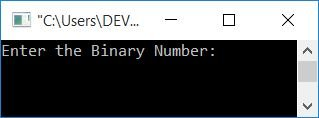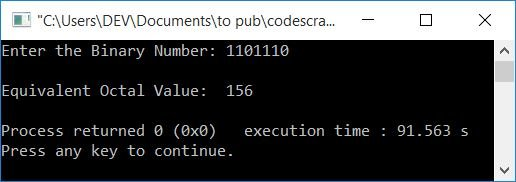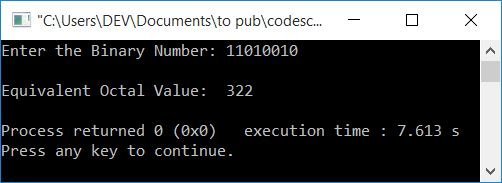# C++ Program to Convert Binary to Octal

In this article, you will learn and get code for binary to octal conversion in C++. The program is written both with and without the use of functions.

But before starting the program, if you are not aware of the steps and formula used for the conversion, you can refer to Binary to Octal. You will find everything you require there.

## Binary to Octal in C++

To convert a binary number to octal in C++ programming, you have to ask the user to enter any number in binary. and then convert it into its equivalent octal value as shown in the program given below.

The question is: write a program in C++ that receives any binary number and prints its equivalent octal value. Here is its answer:

```#include<iostream>
using namespace std;
int main()
{
int binaryNum, octalDigit=0, octalNum;
int i=0, mul=1, chk=1, rem;
cout<<"Enter the Binary Number: ";
cin>>binaryNum;
while(binaryNum!=0)
{
rem = binaryNum%10;
octalDigit = octalDigit + (rem*mul);
if(chk%3==0)
{
octalNum[i] = octalDigit;
mul = 1;
octalDigit = 0;
chk = 1;
i++;
}
else
{
mul = mul*2;
chk++;
}
binaryNum = binaryNum/10;
}
if(chk!=1)
octalNum[i] = octalDigit;
cout<<"\nEquivalent Octal Value:  ";
for(i=i; i>=0; i--)
cout<<octalNum[i];
cout<<endl;
return 0;
}```

This program was build and run under Code::Blocks IDE. Here is its sample run:Now supply the binary number input, say 1101110, and press the ENTER key to print its equivalent value in octal, as shown in the snapshot given below:Here is another sample run with user input as 11010010:The dry run of the above program with user input as 1101110 goes like this:

• Initial values, i = 0, mul = 1, chk = 1, octalDigit = 0.
• When the user enters a binary number, say 1101110, then it gets initialized to binaryNum. Therefore binaryNum=1101110
• Now, because the condition (of the while loop) is binaryNum!=0 or 1101110!=0, evaluates to be true, therefore program flow goes inside the loop.
• Inside the loop, all the statements gets executed one by one, starting from the very first one.
• In other words, binaryNum%10 or 1101110%10 or 0 is initialized to rem. So rem=0.
• octalDigit + (rem*mul) or 0 + (0*1) or 0 is set to octalDigit. So octalDigit=0.
• Now that the condition of the if statement, that is, chk%3==0 or 1%3==0 or 1==0, evaluates to be false, the program does not go inside the if's body, rather it goes to its else's body.
• Inside the else's body, mul*2 or 1*2 or 2 gets initialized to mul. Now mul=2
• And the value of chk gets incremented. Now chk=2
• Finally, the while loop's final statement is executed, and binaryNum/10 or 1101110/10 or 110111 is initialized to binaryNum. Now binaryNum=110111
• Now the program flow goes back and evaluates the condition of the while loop again with the new value of binaryNum.
• The second time, too, the condition evaluates to be true, so the program flow again goes inside the loop.
• This process continues until the condition of the while loop evaluates to be false. That is, the value of binaryNum becomes equal to 0.
• In this way, here are the values after each evaluation of the while loop:
1. rem=0, octalDigit=0, mul=2, chk=2, binaryNum=110111
2. rem=1, octalDigit=2, mul=4, chk=3, binaryNum=11011
3. rem=1, octalDigit=6, octalNum=6, mul=1, octalDigit=0, chk=1, i=1, binaryNum=1101. The condition of if also evaluates to be true at this point.
4. rem=1, octalDigit=1, mul=2, chk=2, binaryNum=110
5. rem=0, octalDigit=1, mul=4, chk=3, binaryNum=11
6. rem=1, octalDigit=5, octalNum=5, mul=1, octalDigit=0, chk=1, i=2, binaryNum=1. Once again, the condition of the if evaluates to be true.
7. rem=1, octalDigit=1, mul=2, chk=2, binaryNum=0
• Because the value of binaryNum is 0 now, the condition of the while loop evaluates to be false, so the while loop evaluation gets ended.
• Now the condition of if, that is, chk!=1, gets evaluated. Because the condition chk!=1 or 2!=1 evaluates to true, the value of octalDigit or 1 is set to octalNum.
• Using the for loop, the value of octalNum[] is now printed from the last to the 0th index.
• That is why the value of octalNum gets printed first. Following that, octalNum and octalNum are printed. That equals or prints 156 as output.

### Binary to octal in C++, using a user-defined function

Let's create another program that does the same job using a user-defined function, BinaryToOctal(). This function receives a binary number as its argument and converts it into its equivalent octal value.

```#include<iostream>
using namespace std;
void BinaryToOctal(int);
int i=0, octalNum;
int main()
{
int binaryNum;
cout<<"Enter any Binary Number: ";
cin>>binaryNum;
BinaryToOctal(binaryNum);
cout<<"\nEquivalent Octal Value: ";
for(i=i; i>=0; i--)
cout<<octalNum[i];
cout<<endl;
return 0;
}
void BinaryToOctal(int binaryNum)
{
int octalDigit=0, mul=1, chk=1, rem;
while(binaryNum!=0)
{
rem = binaryNum%10;
octalDigit = octalDigit + (rem*mul);
if(chk%3==0)
{
octalNum[i] = octalDigit;
mul = 1;
octalDigit = 0;
chk = 1;
i++;
}
else
{
mul = mul*2;
chk++;
}
binaryNum = binaryNum/10;
}
if(chk!=1)
octalNum[i] = octalDigit;
}```

It will produce the same output as the previous program. You can also convert binary to octal in an indirect way. That is, first binary to decimal and then decimal to octal.

#### The same program in different languages

C++ Quiz

« Previous Program Next Program »